# Margins

To view a deliverable’s margin data and statistics, click it’s margin gauge: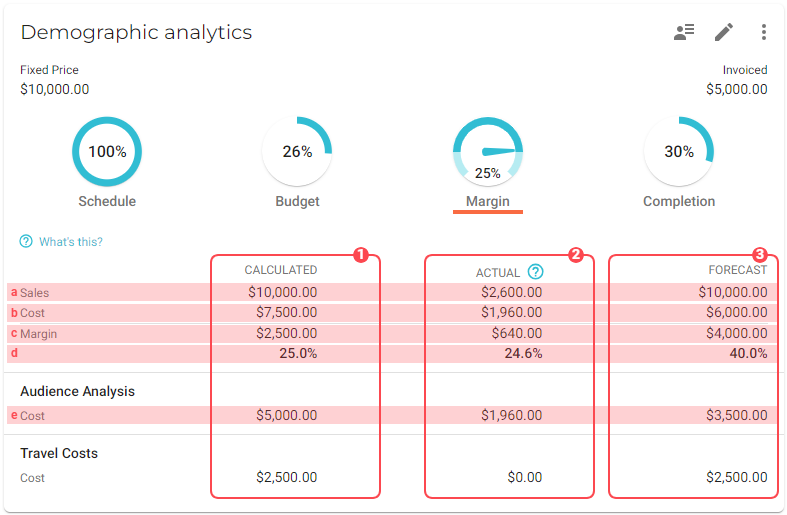The information that displays includes the following: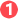The calculated activity, or other costs, such as external services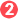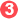## Calculated margin

The calculated margin (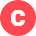) is calculated depending on the invoicing method:

• Time & Material: The total amount of calculated costs divided by the calculated sales (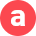).

• Fixed Price: The total amount of calculated costs divided by the total value of the invoicing schedule plus the amounts from manually created invoices().

• Subscription: The total amount of calculated costs divided by the order value of the deliverable ().

Calculated costs (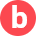) are based on the calculated activities of the deliverable. In this example there are two activities calculated:

Activity Calculated cost rate Hours calculated Total costs

Developing

\$100

200

\$20,000

Design

\$90

100

\$9,000

Total costs

The actual cost () of an activity is displayed beneath it.

## Actual margin

A deliverable’s actual margin is based on its actual costs and actual sales.

### Actual costs

Actual costs are based on all hours spent on the deliverable. The total number of hours spent is multiplied by the cost rate of the employee who submitted the spent hours.

The following example shows how the actual costs are calculated. In this situation, two employees tracked time on a fixed price deliverable:

Employee Cost rate employee Activity Hours spent Total costs

Mikey Johnson

\$90

Developing

32

\$2,880

William Smith

\$100

Design

12

\$1.200

\$4,080

### Actual sales

Since the revenue for fixed price deliverables is based on the invoice schedule, the total amount of all the billing periods is used to calculate the actual sales. If someone created an invoice manually, that invoice amount will also be added to the total.

To have a realistic actual sales value, VOGSY calculates the actual margin in proportion. How to determine the proportion can be configured in the Project settings.

There are three options to choose from:

• Schedule: The percentage of the schedule is used. For example: If 25% of the time is passed, 25% of the total amount of the invoice schedule will be used.

• Budget: The percentage of budget spent is used. For example: when 30% of the budget is used, 30% of the total amount of the invoice schedule is used for calculating the actual margin.

• Completion: The percentage of completion determines the actual sales. The completion can be set manually by tapping on the completion indicator. When the deliverable has a completion percentage of 75%, the actual sales will be 75% of the total amount of the invoice schedule.

The default setting is ‘Completion’.

Based on the above example this would be the calculation of the actual margin, when the total amount of the invoicing schedule is \$37,500 and the completion is 15%:

 Actual costs Hours spent x cost rate employee \$4,080 Actual sales Invoicing schedule (\$37,500) x Completion (15%) \$5,625 Actual margin (\$) Actual sales - Actual costs \$1,545.00 Actual margin (%) Actual margin (\$) / Actual sales 27.5 %